BSc Industrial Eng. | #MachineLearning #ComputerVision #DeepLearning | BI Developer https://www.linkedin.com/in/keremkargin/

# License Plate Detection with OpenCV — Python

In this blog post, we’ll do a small project with OpenCV. In this project, I’ll try to explain how we can detect the vehicle's license plate from a vehicle image. The content of the blog post will be as follows:

• Why is it necessary to detect the license plate?

You can follow me to learn and review more Computer Vision apps like this one. Let’s start.

You can follow my GitHub profile for application-related codes and much more.

## Why is it necessary to detect the license plate?

Plate detection has become one of the most popular…

# Computer Vision Fundamentals and OpenCV Overview

In this blog post, I’ll try to explain the working principle of Computer Vision and, the OpenCV library. Throughout the article I’ll cover:

• What is Computer Vision?

Let’s start step by step. Keep working!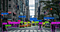Resource: https://medium.com/analytics-vidhya/introduction-to-computer-vision-with-opencv-part-1-3dc948521deb

# What is Computer Vision?

Computer Vision is a workplace that allows us to digitally detect images and perform operations on these images. Computer Vision is an artificial intelligence workspace where we can collect information and extract features by accessing the features of images in digital media. …

# ElasticNet Regression Fundamentals and Modeling in Python

In this blog post, I will first try to explain the basics of ElasticNet Regression. Then, we’ll build the model using a dataset with Python. Finally, we’ll evaluate the model by calculating the mean square error. Let’s get started step by step.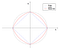Resource: https://www.oreilly.com/library/view/machine-learning-with/9781787121515/5c5ec380-d139-49a5-99b1-3ce32ae5bd6f.xhtml

# What is the ElasticNet Regression?

The main purpose of ElasticNet Regression is to find the coefficients that minimize the sum of error squares by applying a penalty to these coefficients. ElasticNet combines L1 and L2 (Lasso and Ridge) approaches. As a result, it performs a more efficient smoothing process. In another source, it is defined as follows:

Elastic Net first emerged as a…

# Lasso Regression Fundamentals and Modeling in Python

In this blog post, I will first try to explain the basics of Lasso Regression. Then, we’ll build the model using a dataset with Python. Finally, we’ll evaluate the model by calculating the mean square error. Let’s get started step by step.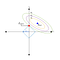Resource: https://waterprogramming.wordpress.com/2017/02/22/dealing-with-multicollinearity-a-brief-overview-and-introduction-to-tolerant-methods/

# What is the Lasso Regression?

The main purpose in Lasso Regression is to find the coefficients that minimize the error sum of squares by applying a penalty to these coefficients. In another source, it is defined as follows:

The “LASSO” stands for Least Absolute Shrinkage and Selection Operator. Lasso regression is a regularization technique. It is used over regression methods for a more…

# Ridge Regression Fundamentals and Modeling in Python

In this blog post, I will first try to explain the basics of Ridge Regression. Then, we’ll build the model using a dataset with Python. Finally, we’ll evaluate the model by calculating the mean square error. Let’s get started step by step.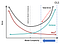Resource: https://medium.com/kaveai/ridge-ve-lasso-regresyonu-temel-matemati%C4%9Fi-ve-python-uygulamas%C4%B1yla-363916e32d8d

# What is the Ridge Regression?

The main purpose of Ridge Regression is, to find the coefficients that minimize the sum of error squares by applying a penalty to these coefficients. Also known as Ridge Regression L2. In another source, it is defined as follows.

Ridge regression is a model tuning method that is used to analyse any data that suffers from multicollinearity. This…

# Multiple Linear Regression Fundamentals and Modeling in Python

In this blog post, first, I’ll try to explain the basics of Multiple Linear Regression. Then, I’ll build the model using a dataset with Python. Finally, I’ll evaluate the model by calculating the mean square error. Let’s get started step by step.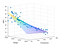Resource: https://medium.com/analytics-vidhya/new-aspects-to-consider-while-moving-from-simple-linear-regression-to-multiple-linear-regression-dad06b3449ff

# What is the Multiple Linear Regression?

The main purpose of Multiple Linear Regression is to find the linear function expressing the relationship between dependent and independent variables. Multiple Linear Regression model has one dependent and more than one independent variable. In another source, it is defined as follows:

Multiple linear regression is used to estimate the relationship between two or more independent variables and

# Simple Linear Regression Fundamentals and Modeling in Python

In this blog post, I will first try to explain the basics of Simple Linear Regression. Then, we’ll build the model using a dataset with Python. Finally, we’ll evaluate the model by calculating the mean square error. Let’s get started step by step.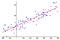Resource: https://en.wikipedia.org/wiki/Linear_regression

# What is the Simple Linear Regression?

Simple Linear Regression is a statistical method that helps us describe and analyze the relationship between two variables, one dependent and one independent. In another source, it is defined as follows:

Simple linear regression is used to estimate the relationship between two quantitative variables. You can use simple linear regression when you want to know:

- How…

# Apache Kafka Architecture - Getting Started with Apache Kafka

In this blog post, I will try to explain what is Apache Kafka, how it works, when to use it, writing data to Kafka, and reading data from Kafka. Enjoyable readings.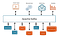Resource: http://www.techmonks.org/kafka-terminology/

Let’s first take a look at the architecture in the past. In the ’90s CLIENT-SERVER architecture was popular. There was a Monolith structure here. In Monolith structure; all structures are included in a single application. Access to the internet increased over time. And version updates have become a constant need. For this reason, the number of people who prefer this structure has decreased over time.

Then Microservices came to…

# NLP: Tokenization, Stemming, Lemmatization and Part of Speech Tagging

In this blog post, I’ll talk about Tokenization, Stemming, Lemmatization, and Part of Speech Tagging, which are frequently used in Natural Language Processing processes. We’ll have information about how to use them by reinforcing them with applications. Enjoyable readings.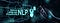Resource: https://www.asksid.ai/resources/what-is-natural-language-processing/

## Tokenization

`Tokenization` is the process of breaking down the given text in natural language processing into the smallest unit in a sentence called a token. Punctuation marks, words, and numbers can be considered tokens. So why do we need `Tokenization`? We may want to find the frequencies of the words in the entire text by dividing the given text into tokens. Then…

# Introduction to Natural Language Processing

In this post, I will try to share the basics of Natural Language Processing, its uses, as well as Text Mining and Natural Language Processing. I intend to mention in a different article than text preprocessing in natural language processing. Enjoyable readings.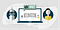Resource: https://aliz.ai/natural-language-processing-a-short-introduction-to-get-you-started/

## What is the Natural Language Processing?

Before I define Natural Language Processing myself, let’s look at how it is defined in several sources.

Natural Language Processing, or NLP for short, is broadly defined as the automatic manipulation of natural language, like speech and text, by software.

In another definition, it is defined as follows;

Natural language processing is a form of artificial intelligence (AI)…